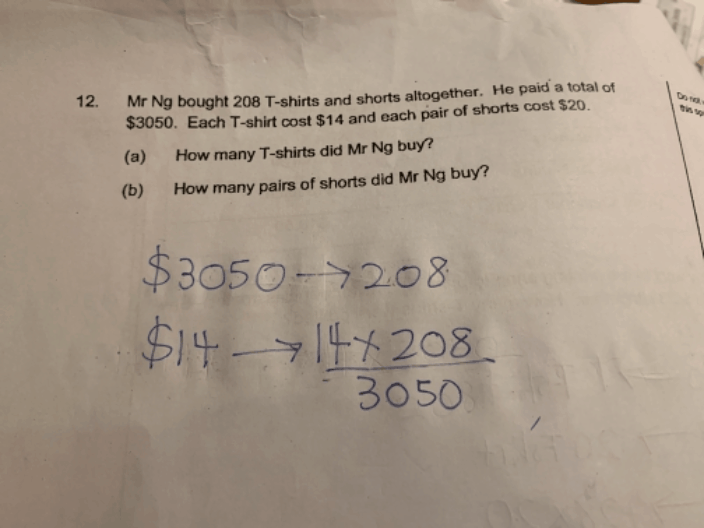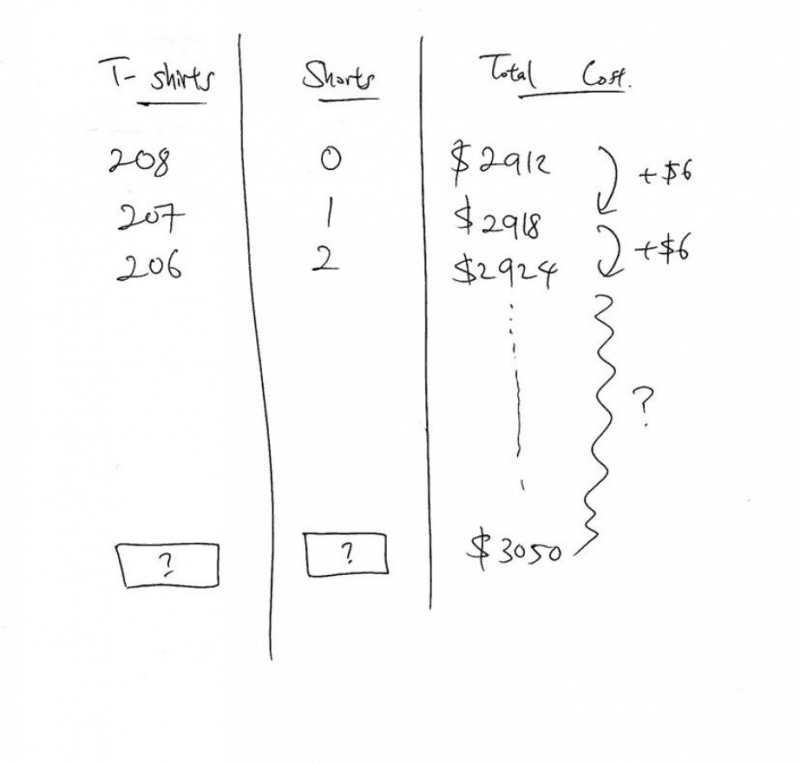# QuestionSource: Nan Hua Primary

1 Answer

# AnswerHi

This is a question that uses this method called the Assumption Method. It is almost like the guess and check method where you will blindly guess and check for the correct answer. But this is more organised; think of it like a more organised guess and check method.

The Assumption Method is named as such because firstly, to solve the question with the method we will assume either all items are T shirts and none are Shorts, or we can assume all are Shorts and none are T shirts. Starting off with either way is perfectly fine.

So let’s say we assume that all the items bought are T shirts and none are Shorts, it would mean that there will be 208 T shirts and 0 shorts. That would cost \$2912.

Next, we will subtract 1 T shirt and add on 1 pair of shorts. Now we will have 207 T shirts and 1 pair of shorts. We will remember to keep the total number of items at 208. That would cost \$2918.

Next, we will again subtract 1 T shirt and add on 1 more pair of shorts. Now we will habe 206 T shirts and 2 pairs of shorts. Again the total number of items must be kept at 208. That would cost \$2924.

If we were to keep on adding one and subtracting the other 1 by 1, we notice that our total cost for the items will keep increasing by \$6 each time. At this point of time, do take note that our “target” value for the total cost of items is \$3050.

So from our starting point of \$2912, we need to reach \$3050. And in between those 2 values is a difference of \$3050-\$2912= \$138.

That would also tell us that we need to take 23 steps from our starting point. Because \$138 divided by \$6 is 23. (remember, each step of adding one and subtracting one will change the value of the total cost by \$6)

Therefore, looking back at our starting point, to find out the number of T shirts that we have, we take 208-23, and that will be 185. We have 185 t shirts.

Looking back at our starting point, to find out the number of pairs of shorts that we have, we take 0+23, and that will be 23. We have 23 pairs of shorts.

To check our answer to see if it tallies,

185 x \$14 = \$2590

23 x \$20 = \$ \$460

\$2590 + \$460 = \$3050

Hope this helps. Feel free to drop me a message if you need more help.

We provide coaching for small, focus groups of students at Future Achievers Hub at Tampines Junction.

Do check out:

www.futureachievers.com.sg

or call us at +65 83325521,

for more info and sign up for Free Classes now!

0 Replies 1 Like